• ## Matplotlib

万次阅读 多人点赞 2018-08-23 23:28:21
该格式化字符串的字母和符号均来自于MATLAB，由一个代表颜色的字符串和一个代表线型的字符串组合而成。默认使用的格式化字符串是’b-‘（表示蓝色实线）。   如果想要使用红色的圆点来绘制上例中的图形，你可以...
官网帮助文档地址：https://matplotlib.org/tutorials/introductory/pyplot.html
Pyplot简介
matplotlib.pyplot 是一个命令风格的函数集合，这使得 matplotlib 工作起来和MATLAB很相似。每一个 pyplot 函数都会使图形发生一些变化，例如：创建一幅图形、在一幅图中创建一个绘图区域、在绘图区域中绘制一些线、使用标签对图形进行修饰，等等。在matplotlib.pyplot中，各种各样的状态通过调用不同的函数被保存下来，因此，matplotlib 能够持续地对例如：当前的图形和绘图区域、并将绘图函数作用到当前轴上（请注意，这里和文档中大多数地方的“轴”指的是图形的坐标系部分，而不是严格数学术语中的多个轴）。            使用 pyplot 生成一个可视化效果非常快：
import matplotlib.pyplot as plt
plt.plot([1,2,3,4])
plt.ylabel('some numbers')
plt.show()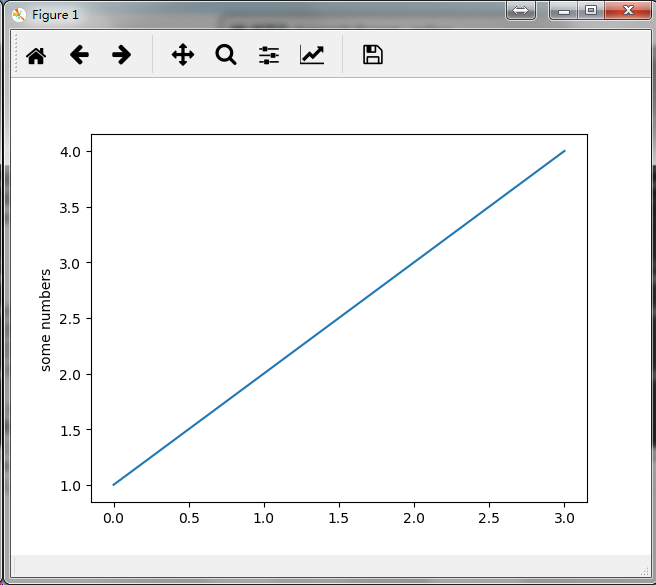你可能会觉得很奇怪：为什么X轴取值的范围是 0~3，而Y轴取值的范围是1~4 ？
这是因为：如果你只提供 了一个列表或者数组作为 plot() 命令的参数，Matplotlib 就会把这个序列当作是Y轴上的取值，并自动地为你生成 X轴上的值。由于在python中范围是从0开始的，因此在默认情况下，X轴向量会与Y轴向量的长度相同但其取值会从0开始，正如我们所看到的X轴的数据是[0,1,2,3]。            plot() 是一个多功能的命令，能够接收任意数量的参数，例如：你可以使用如下命令来绘制与X相对的Y的图形。
plt.plot([1, 2, 3, 4], [1, 4, 9, 16])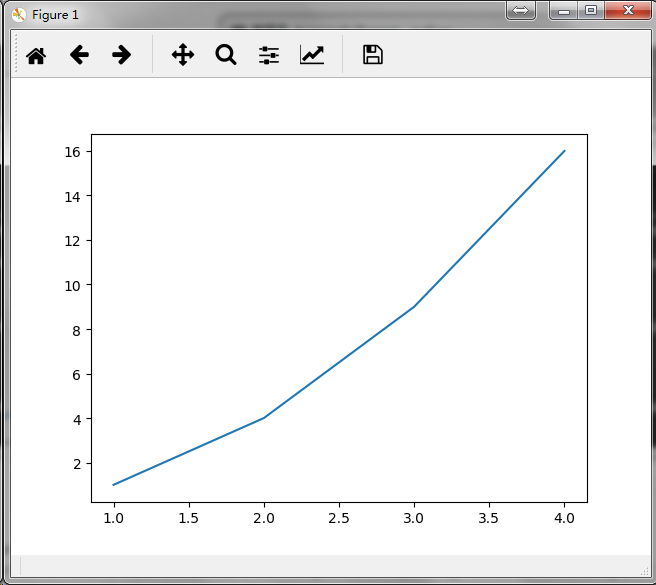格式化图形样式
plot() 还为每一对参数X和Y提供了一个可选的第三个格式化字符串参数用来指定图形的颜色和线型。该格式化字符串的字母和符号均来自于MATLAB，由一个代表颜色的字符串和一个代表线型的字符串组合而成。默认使用的格式化字符串是’b-‘（表示蓝色实线）。             如果想要使用红色的圆点来绘制上例中的图形，你可以这么写：
plt.plot([1, 2, 3, 4], [1, 4, 9, 16], 'ro')
plt.axis([0, 6, 0, 20])
plt.show()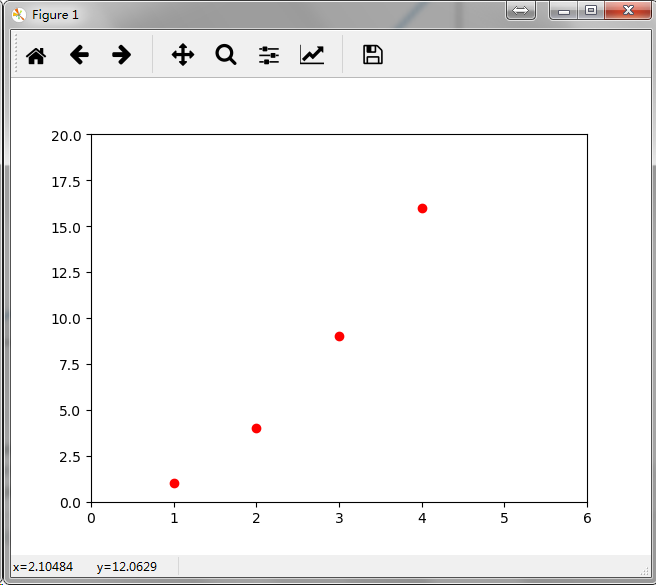你可以查看 plot() 的文档来获取关于线的样式和格式化字符串的完整列表。             上例中的 axis() 命令接收了 [xmin, xmax, ymin, ymax] 列表作为参数来指定了各个轴的视口大小。但如果 Matplotlib 被限制成只能和 list 一起使用 ，那么它在数值处理上将显得几乎毫无用处 。通常，我们都会使用 numpy 数组，事实上，所有的序列都会在 Matplotlib 内部被转化成 numpy 数组。下面的例子展示了如何在一条命令里使用  numpy 数组绘制出几条样式不同的线：
import numpy as np

# evenly sampled time at 200ms intervals
t = np.arange(0., 5., 0.2)

# red dashes, blue squares and green triangles
plt.plot(t, t, 'r--', t, t**2, 'bs', t, t**3, 'g^')
plt.show()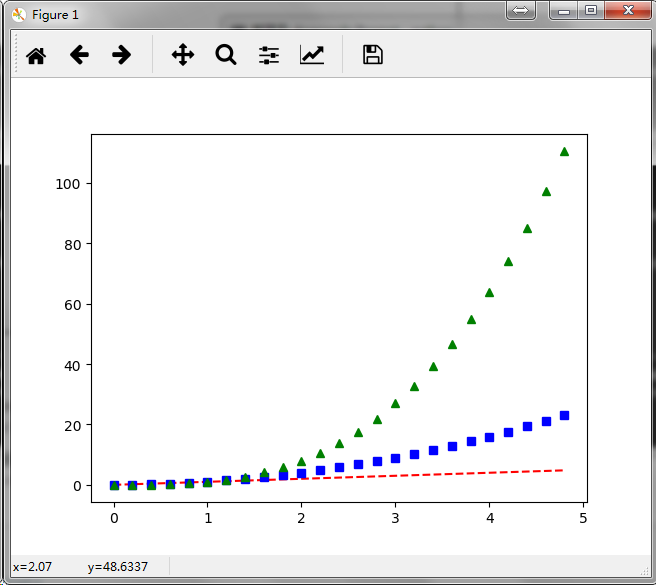补充：在 plot()  中，格式化字符串由颜色、标识符和线型三部分组成，定义格式如下：
fmt = '[color][marker][line]'
其中，支持的颜色缩写有如下几个：
charactercolor'b'blue'g'green'r'red'c'cyan'm'magenta'y'yellow'k'black'w'white
标识符定义如下表所示：
characterdescription'.'point marker','pixel marker'o'circle marker'v'triangle_down marker'^'triangle_up marker'<'triangle_left marker'>'triangle_right marker'1'tri_down marker'2'tri_up marker'3'tri_left marker'4'tri_right marker's'square marker'p'pentagon marker'*'star marker'h'hexagon1 marker'H'hexagon2 marker'+'plus marker'x'x marker'D'diamond marker'd'thin_diamond marker'|'vline marker'_'hline marker
可以选用的线型有以下四个：
characterdescription'-'solid line style'--'dashed line style'-.'dash-dot line style':'dotted line style
以下是几个格式化字符串的示例：
'b'    # blue markers with default shape
'ro'   # red circles
'g-'   # green solid line
'--'   # dashed line with default color
'k^:'  # black triangle_up markers connected by a dotted line
使用关键字绘图
Matplotlib 允许用户提供带有关键字的数据对象作为参数，一旦提供了带有关键字的数据，就能够直接使用关键字来代替这些变量去生成图形。            我们可以使用某些命令来获取到这种格式的数据，例如：numpy.recarray 和 pandas.DataFrame  。
data = {'a': np.arange(50),
'c': np.random.randint(0, 50, 50),
'd': np.random.randn(50)}
data['b'] = data['a'] + 10 * np.random.randn(50)
data['d'] = np.abs(data['d']) * 100

plt.scatter('a', 'b', c='c', s='d', data=data)
plt.xlabel('entry a')
plt.ylabel('entry b')
plt.show()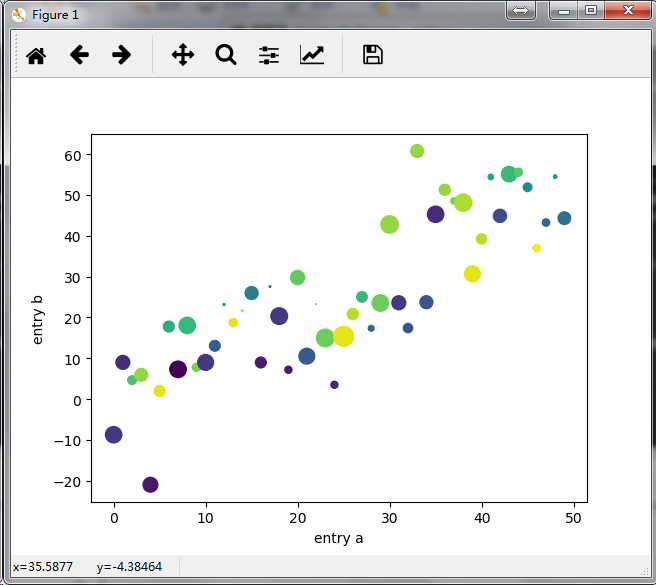使用分类变量绘图
使用分类变量来创建图形也是可以的，Matplotlib 允许用户直接把分类变量传递给多个绘图函数，例如：
names = ['group_a', 'group_b', 'group_c']
values = [1, 10, 100]

plt.figure(1, figsize=(9, 3))

plt.subplot(131)
plt.bar(names, values)
plt.subplot(132)
plt.scatter(names, values)
plt.subplot(133)
plt.plot(names, values)
plt.suptitle('Categorical Plotting')
plt.show()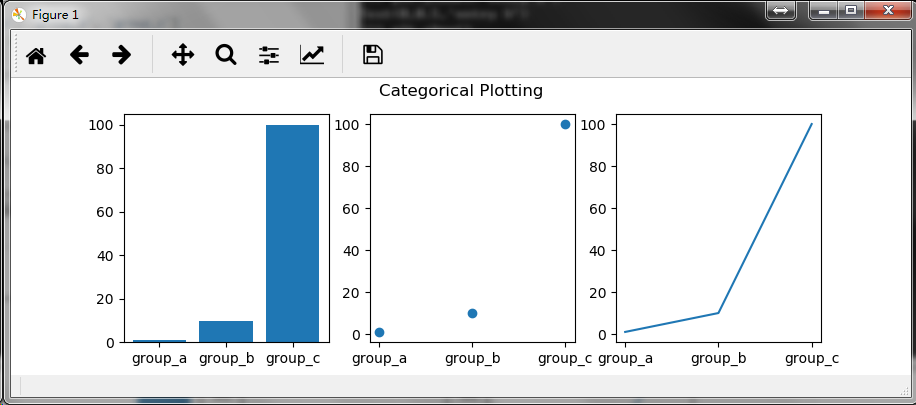控制线的属性
线的属性有很多，详情参见  matplotlib.lines.Line2D ，例如你可以设置：线宽、虚线和抗锯齿效果，等等。            我们可以通过以下几种途径对线的属性进行设置：            使用关键字参数
plt.plot(x, y, linewidth=2.0)
使用2D 线实例的set方法            plot() 命令会返回一个线对象的列表，例如：line1, line2 = plot(x1, y1, x2, y2)。在下面的代码中，我们将假设我们只有一条线即plot() 命令返回的线列表的长度为 1 。我们使用 line, 来获取列表中的第一个元素。
line, = plt.plot(x, y, '-')
line.set_antialiased(False) # turn off antialising
使用setp()命令            下面示例一下如何使用MATLAB风格的命令对一个线列表的多个属性进行设置。很明显地，setp() 能够作用于一个对象列表或者是一个单独的对象。你既可以使用Python 的关键字作为参数也可以使用MATLAB风格的string/value 对作为参数：
lines = plt.plot(x1, y1, x2, y2)
# use keyword args
plt.setp(lines, color='r', linewidth=2.0)
# or MATLAB style string value pairs
plt.setp(lines, 'color', 'r', 'linewidth', 2.0)
2D 线实例的可用属性见下表：
PropertyValue Typealphafloatanimated[True | False]antialiased or aa[True | False]clip_boxa matplotlib.transform.Bbox instanceclip_on[True | False]clip_patha Path instance and a Transform instance, a Patchcolor or cany matplotlib colorcontainsthe hit testing functiondash_capstyle['butt' | 'round' | 'projecting']dash_joinstyle['miter' | 'round' | 'bevel']dashessequence of on/off ink in pointsdata(np.array xdata, np.array ydata)figurea matplotlib.figure.Figure instancelabelany stringlinestyle or ls[ '-' | '--' | '-.' | ':' | 'steps' | ...]linewidth or lwfloat value in pointslod[True | False]marker[ '+' | ',' | '.' | '1' | '2' | '3' | '4' ]markeredgecolor or mecany matplotlib colormarkeredgewidth or mewfloat value in pointsmarkerfacecolor or mfcany matplotlib colormarkersize or msfloatmarkevery[ None | integer | (startind, stride) ]pickerused in interactive line selectionpickradiusthe line pick selection radiussolid_capstyle['butt' | 'round' | 'projecting']solid_joinstyle['miter' | 'round' | 'bevel']transforma matplotlib.transforms.Transform instancevisible[True | False]xdatanp.arrayydatanp.arrayzorderany number
如果你想要获取可供设置的线属性的列表，你可以直接调用 setp() 命令并传入一条线或者传入一个线列表作为唯一参数。
lines = plt.plot([1, 2, 3])

plt.setp(lines)
alpha: float
animated: [True | False]
antialiased or aa: [True | False]
...snip
使用多图形和多轴
MATLAB和 pyplot 都有当前图形和当前轴的概念，所有的绘图命令都会被应用到当前轴上。在 Matplotlib 中，gca() 命令用来获取当前轴（一个 matplotlib.axes.Axes 实例），gcf() 命令用来获取当前图形（一个 matplotlib.figure.Figure 实例）。通常情况下，你并不需要考虑这些，因为 Matplotlib 会在后台对它们进行自动处理。            下面是一个创建了两个子图形的示例脚本：
def f(t):
return np.exp(-t) * np.cos(2*np.pi*t)

t1 = np.arange(0.0, 5.0, 0.1)
t2 = np.arange(0.0, 5.0, 0.02)

plt.figure(1)
plt.subplot(211)
plt.plot(t1, f(t1), 'bo', t2, f(t2), 'k')

plt.subplot(212)
plt.plot(t2, np.cos(2*np.pi*t2), 'r--')
plt.show()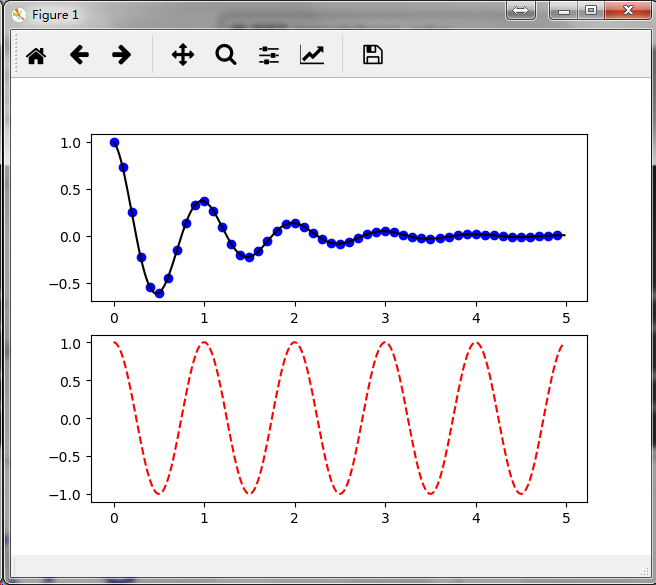figure()命令在这儿是一个可选项，因为默认创建的就是 figure(1) ，同样地，在没有手动地指定任何轴的情况下，将默认创建 subplot(111) 。subplot() 命令需要指定行数、列数、图形编号，其中图形编号的取值范围为（1 ~ 行数*列数）。如果行数*列数<10，参数之间的逗号可以省略。因此，subplot(211) 和 subplot(2, 1, 1) 的作用是一样的。
你可以创建任意数量的子图形或者坐标系。如果你想要手动去放置一个坐标系可以使用 axes() 命令，并通过  axes([left, bottom, width, height]) 的四个参数（ 0 ~ 1 的小数）来指定坐标系的位置和大小。
手动设置坐标系示例：https://matplotlib.org/gallery/subplots_axes_and_figures/axes_demo.html            绘制多个子图形示例：            https://matplotlib.org/gallery/subplots_axes_and_figures/subplot_demo.html            https://matplotlib.org/gallery/subplots_axes_and_figures/subplots_demo.html            你可以通过多次调用figure() 方法并传入一个递增的图形编号来创建多个图形。当然，只要你喜欢每一个图形也可以包含多个子图形或者坐标系：
import matplotlib.pyplot as plt
plt.figure(1)                # the first figure
plt.subplot(211)             # the first subplot in the first figure
plt.plot([1, 2, 3])
plt.subplot(212)             # the second subplot in the first figure
plt.plot([4, 5, 6])

plt.figure(2)                # a second figure
plt.plot([4, 5, 6])          # creates a subplot(111) by default

plt.figure(1)                # figure 1 current; subplot(212) still current
plt.subplot(211)             # make subplot(211) in figure1 current
plt.title('Easy as 1, 2, 3') # subplot 211 title
plt.show()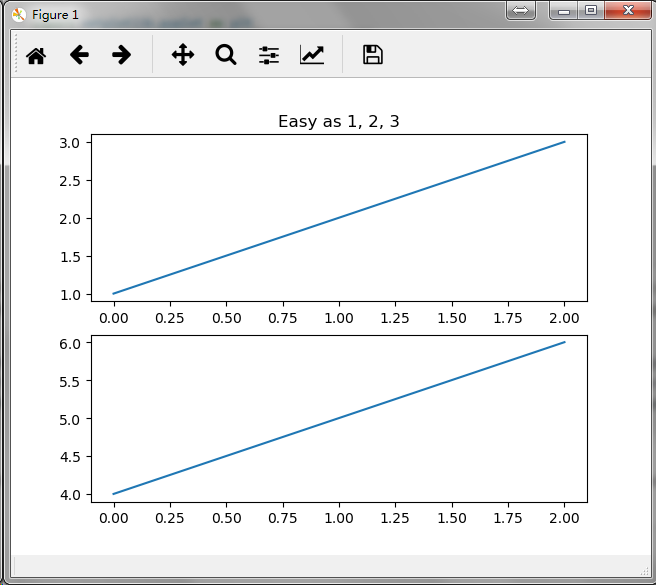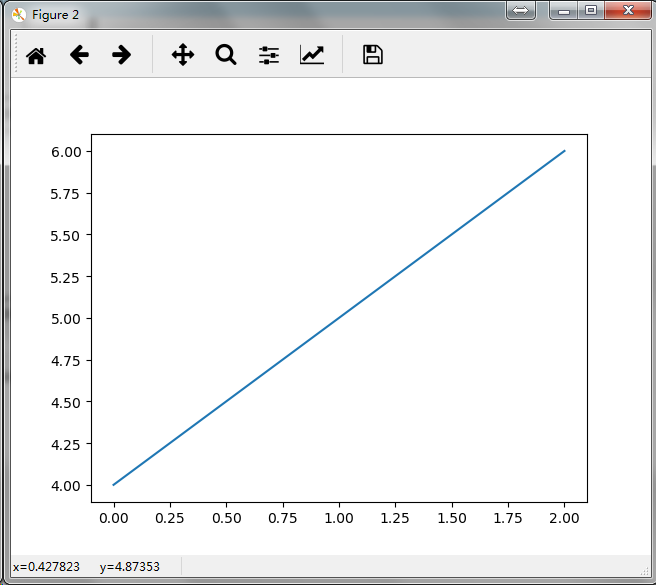你可以使用 clf() 命令来清除当前图形或者使用 cla() 命令来清除当前坐标系。 如果你觉得由后台帮你维护图形状态（指当前的图像，图形和坐标系）这种方式比较烦人，无须绝望：这只是对面向对象API的一个瘦状态封装，你可以把它替换掉（参见 Artist tutorial ）。             如果你是在创建多个图形，你还需要注意另外一件事：图形所占用的内存直到你调用 close() 显式地将其关闭才会全部释放，如果只删除掉图形的引用或者通过窗口管理器销毁掉屏幕上的图像展示窗口，图形所占用的内存是不能完全被释放的，因为pyplot 在 close() 命令被调用之前仍会对其内部引用进行维护。
使用文本
使用 text() 命令可以在图形的任意位置添加文本，xlabel()、ylabel()、title() 用来在指定位置添加文本（更多详细案例参见 Text in Matplotlib Plots ）。
mu, sigma = 100, 15
x = mu + sigma * np.random.randn(10000)

# the histogram of the data
n, bins, patches = plt.hist(x, 50, density=1, facecolor='g', alpha=0.75)

plt.xlabel('Smarts')
plt.ylabel('Probability')
plt.title('Histogram of IQ')
plt.text(60, .025, r'$\mu=100,\ \sigma=15$')
plt.axis([40, 160, 0, 0.03])
plt.grid(True)
plt.show()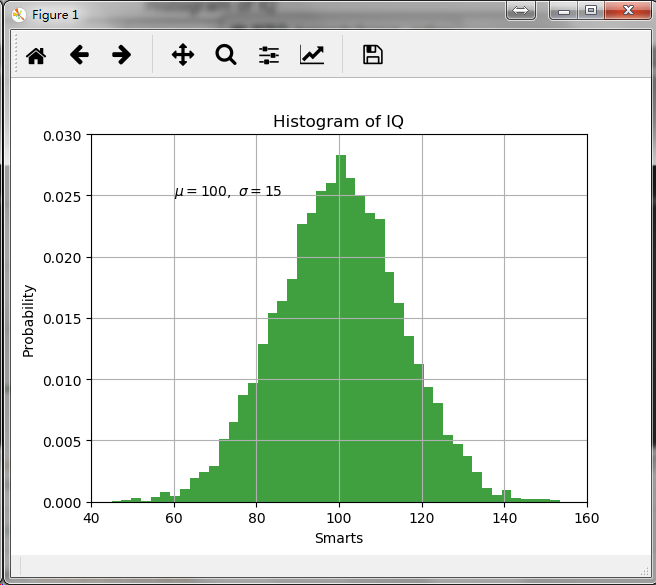所有的文本命令都会返回一个  matplotlib.text.Text  实例，跟前面线的属性设置一样，你可以往文本命令中传入关键字参数或者使用 setp() 命令来自定义文本的属性。
t = plt.xlabel('my data', fontsize=14, color='red')
关于文本的属性的更多细节请参见：https://matplotlib.org/tutorials/text/text_props.html
在文本中使用数学表达式
• ## pyecharts 组合图

千次阅读 2020-06-14 23:39:50
from pyecharts.charts import Bar, Line, Grid from pyecharts import options # 1. 准备数据 cate = ["湖北", "四川", "重庆", "河北", "云南"] Confirmed_diagnosis_data = [450, 300, 232, 224, 144] death_...
• import tushare as ts from pyecharts.faker import Faker from pyecharts import options ...from pyecharts.charts import Bar,Kline,Line,Grid from pyecharts.globals import ThemeType df=ts.get_hist_da...pyecharts 学习笔记
• 最近面试遇到一个问题就是，Spark实现对统计 “年级, 班级, 学生, 分数” 的Top3； 除了此外问题还有： 每个班级分数前三名同学的名字以及分数； 各省指标数量前三的市的名字； 测试数据： 一年级、一...Spark Scala
• 最近需要做个商品列表，在手机屏幕不太一样的市场里，如何做到列表中刚刚好显示2行，偶然间发现淘宝的手机版有用到-webkit-line-clamp来实现这种效果 ...为了实现该效果，它需要组合其他外来的WebK...
• ## C++ 面试题大全

千次阅读 多人点赞 2017-05-31 09:42:54
1.new、delete、malloc、free关系 delete会调用对象的析构函数,和new对应，free只会释放内存，new调用构造函数。malloc与free是C++/C语言的标准库函数，new/delete是C++的运算符。它们都可用于申请动态内存和释放...C++ 面试题大全
• 树导航效果图：组合模式的描述：将对象组合成树形结构以表示“部分-整体”的层次结构，组合模式使得用户对单个对象和组合对象的使用具有一致性。我们把部分用Leaf表示， 把整体用Composite表示。组合模式是有一定...
• 一、鼎捷易拓TIPTOP GP简介： 易拓ERP(TOP GP ERP)是以大型集团化企业为主要客户对象的ERP解决方案，并以“集团统筹、全球掌控”为核心理念。迄今已经协助数千家企业信息化实施，其范围覆盖快速成长型企业、大中...
• 每条背景的组合 */ private ArrayList<BgColor> getBg () { ArrayList<BgColor> bgList = new ArrayList(); bgList.add( new BgColor( 10 , 20 , Color.YELLOW)); //参数信息：纵坐标从0到20设置颜色...android
• 绘制折线图 #绘制 line_ensur=Line() 1、 绘制 确诊数折线图 #添加横轴 line_ensur.add_xaxis(x_data) #添加纵轴 line_ensur.add_yaxis(series_name='确诊数', y_axis=ensue_num, color='#4b0101', label_opts=opts....数据分析 数据可视化 python
• ## ul li span 小组合

千次阅读 2014-03-25 14:30:07
line-height: 1.35em;  padding: 4px 20px; /* 内部填充的距离 10px 10px 10px 55px*/  text-decoration: none;  white-space: nowrap; /* 对于文本内的空白处，不会换行，文本会在在同一行上继续，...
• from pyecharts import options as ...from pyecharts.charts import Bar, Line from pyecharts.faker import Faker # 数据 v1 = [2.0, 4.9, 7.0, 23.2, 25.6, 76.7, 135.6, 162.2, 32.6, 20.0, 6.4, 3.3] v2 = [2...
• pyecharts/charts/composite_charts/包中的四个模块分别定义了组合图表类，其中pyecharts/charts/composite_charts/timeline.py模块只定义了时间线类Timeline。 Timeline类继承自图表基类Base，作用为在一个echarts...pyecharts charts timeline...